Publication date: 03/23/2021

# Row State Functions

There are six characteristics that rows in a data table can have: selected, hidden, excluded, labeled, colored, and marked. If you give rows one or more of these characteristics and then create row state data table columns, you can then create a formula that computes and saves row state conditions. (See Column Properties in JMP, and Store Information in Row State Columns.)This formula processes row state data just as it would process character and numeric data.

See Row State Functions in the JSL Syntax Reference for more information about syntax.

Note: A row can be assigned any combination of row states; a row state column can have multiple row states as a value.

Table A.2 describes the type of argument each Row State function requires and what each returns.

Table A.2 Row State Functions

Function Name

Argument Type Required

What the Function Returns (Your Column Data Type Should be This Type)

Row State

none

row state of current row

As Row State

numeric

all row states of current row

Combine States

multiple row state arguments

multiple row state assignments

Excluded State

positive integer or zero

row state-excluded or not excluded

Hidden State

positive integer or zero

row state-hidden or not hidden

Labeled State

positive integer or zero

row state-labeled or not labeled

Color State

integer or color name or {red, green, blue}

row state color

Marker State

integer or character

row state marker

Selected State

positive integer or zero

row state-selected or not selected

Hue State

integer

row state hue

Shade State

integer 1-5

row state intensity

Excluded

Row State() or row state column

integer 0 (not excluded) or 1 (excluded)

Hidden

Row State() or row state column

integer 0 (not hidden) or 1 (hidden)

Labeled

Row State() or row state column

integer 0 (not labeled) or 1 (labeled)

Color Of

Row State() or row state column

color map integer

Marker Of

Row State() or row state column

marker map integer

Selected

Row State() or row state column

integer 0 (not selected) or 1 (selected)

#### Row State

Returns the active row state condition of the current row as true or false. You can use this function to conveniently write conditional clauses that depend on the status of the current row. For example, Figure A.15 assigns a 1 to rows that are currently selected and labeled and 0 otherwise.

Figure A.15 Row State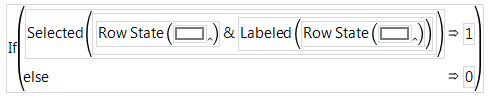#### As Row State

Converts a numeric argument to a row state or set of row state conditions. Row states are stored internally in JMP as a 16-bit number, with each bit assigned to represent one of the possible row states as illustrated in Figure A.3. For example, the binary representation of 1327 is 0000010100101111. As Row State(1327) would therefore set the row state as selected, excluded, hidden, labeled, with marker 2 and color 10.

Table A.3 Row States Stored as 16-Bit Numbers: Each Bit Represents a Row State

Bit

Row State

0

Not selected (0) or Selected (1)

1

Unexcluded (0) or Excluded (1)

2

Unhidden (0) or Hidden (1)

3

Unlabeled (0) or Labeled (1)

4-7

Marker

8-14

Color

#### Combine States

Generates a row state combination with two or more arguments. Use the insert button (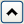) on the Formula Editor keypad or type a comma to add arguments to the Combine States function. The currently selected expression becomes the first argument when you select Combine States. Replace each argument with an expression that evaluates to a row state. This formula:

`Combine States(`
`	Selected State( Modulo( Row(), 2 ) ),`
`	Labeled State( Modulo( Row() + 1, 2 ) ) );`

alternately labels or selects each row in the calculated row state column. The Selected State and Labeled State functions are defined later in this section. Use the insert () and delete (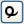)buttons on the Formula Editor keypad to add more arguments or remove unwanted arguments.

If you include conflicting row states in a combination, the results are unpredictable.

#### Excluded State

Interprets a numeric argument as true or false. When an argument evaluates as true, the Excluded State function assigns the excluded condition as the value of the column for that row. For example, Excluded State(Modulo(Row(),2)) assigns the excluded row state as the value of the row state column for each odd numbered row.

#### Hidden State

Assigns the hidden row state condition when its argument is greater than zero. If the argument is zero, the value in the column for that row is not hidden.

#### Labeled State

Gives the labeled row state condition when its argument is greater than zero. If the argument is zero the row value in the column for that row is not labeled.

#### Color State

Returns the color from the JMP color map that corresponds to its integer argument. JMP colors are numbered 0 through 84. Zero maps to black.

#### Marker State

Returns markers from the JMP marker map that correspond to its integer argument. JMP markers are numbered 0 through 16. The formula Marker State(Row()) assigns all the row state markers in a repeating sequence determined by the current row number to the calculated row state column. A row state column can have multiple row states as a value.

#### Selected State

Gives the selected row state condition when its argument is greater than zero. If the argument is zero, the value in the column for that row is not selected.

#### Hue State

Returns the color from the JMP hue map that corresponds to its integer argument. JMP hues are numbered 0 through 11 but larger integers are treated as modulo 12. The Hue State function does not map to black, gray, or white. A hue of zero maps to red, and a hue of 11 maps to magenta. The formula in Figure A.16 assigns row state colors in a chromatic spread based on the value of z. The Hue State function is used with a row state data type column.

Figure A.16 Example of Hue State Function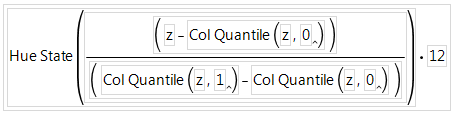#### Shade State

Assigns five shade levels to a color or hue. A shade of –2 is darkest and shade of +2 is lightest. A shade of zero is a pure color. The formula in Figure A.17 assigns shade values based on the value of z.

Figure A.17 Example of Shade State Function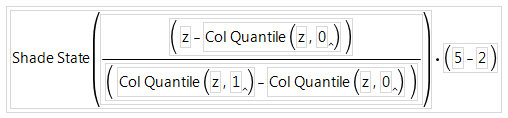To assign all shades of all the colors in the colors palette, you need to use the Hue State and Shade State assignments together. The formula in Figure A.18 uses the Combine States function described at the beginning of this section. The first argument in the Combine States function is the Hue State formula shown previously, and the second argument is the Shade State formula. In addition, the Marker State function with an argument of 2 assigns the X marker to each row, and the Selected State function with an argument of 1 selects each row.

Figure A.18 Combine States Example For Using Both Hue State and Row State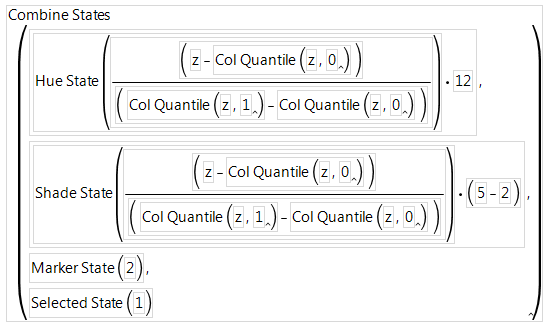#### Excluded, Hidden, Labeled, and Selected

Accepts a row state expression argument (row state column or row state constant) that evaluates as either 1 or 0 (true or false). These characteristics are inactive by default. Often, the Row() function is the argument, which detects the active row state condition of each row. For example, in Figure A.19, the formula assigns 99 whenever a row is actively selected, and 0 otherwise. Note that this formula is used in a column that has a numeric data type.

Figure A.19 Example of a Formula Using the Selected Function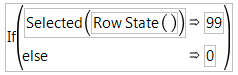The example in Figure A.20 assigns row state conditions to a row state column. The formula for the row state column (in the column called x) checks to determine whether the active row state is either Hidden or Excluded, and if so, assigns the Labeled row state.

Figure A.20 Calculate Row State Information in a Row State Column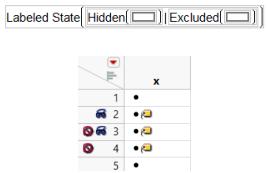#### Color Of

Accepts any row state expression or column, or the Row State() function as its argument. Returns a number from the JMP color map that corresponds to the active color state, or zero if there is no assigned color.

#### Marker Of

Accepts any row state expression or column, or the Row State() function as its argument. Returns a number from the JMP marker map that corresponds to the active marker or zero if there is no assigned marker.

Want more information? Have questions? Get answers in the JMP User Community (community.jmp.com).